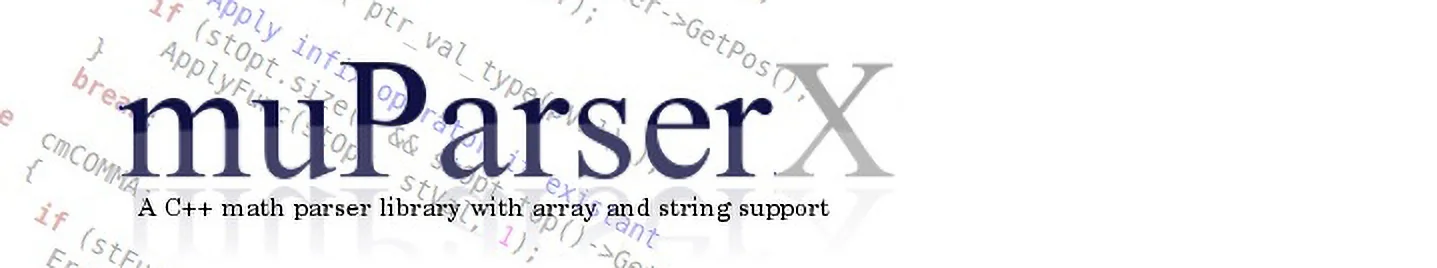# Expression Syntax

## Basic Types

Internally muparserx is using a variant style datatype which can represent values (complex or not), strings and matrices.

### String values

String values can only be used if the parser was initialized with the pckSTRING flag. This is the case when using a default initialized parser instance. When used in a parser expression string values must be enclosed in quotation marks as shown in the folowing example:

``"This is a text"``

muparserx can use the backslash character for escaping special characters. The following escape sequences are supported:

By using the proper escape sequence creating a string containing two lines can be achieved easily:

``"This is the first line\nThis is the second line"``

Concatenating strings can be done with the operator "//":

``"Hello "//"World"``

### Booloean values

The parsing engine supports boolean values. The keywords true and false can be used to assign boolean contant like for instance:

``a=true``

### Numeric values

Internally muparserx does not distinguish between integer and floating point values. Values in exponential format are accepted:

``a=1.67e-4``

### Complex Numbers

When used in complex mode, the parser defines a constant representing the imaginary unit i. This constant can be used exactly like any other variable with the exception that you can omit the '*' between 'i' and values. For instance defining a complex variable a can simply be achieved by using either the short syntax:

``6+9i``

or the long syntax:

``6+9*i``

## Matrices and arrays

In order to use matices muparserx must be initialized with the pckMATRIX flag. This flag will activate the transpose operator and the functions `ones`, `eye` and `zeros` which can be used for creating new matrices on the fly. Matrix and array values are exactly treated like any other value. The only exception is that they support the index operator. The index operator can either be one or two dimensional, depending on the dimension of the parser variable. When using the index operator on matrices the index dimensions are seperated with a comma. The first index refers to the rows of the matrix, the second to the columns.

``m[1,2]=1``

All Matrix and vector indices are zero based!

### Creating Vectors

A simple row vector can be created by using the {...} operator. Currently you can not use any other values than scalars as the arguments to the vector creation operator.

``{1,2,3}``

If you need a column vector it can be transformed easily by using the transpose postfix operator:

``{1,2,3}'``

### Creating Matrices

muparserx provides several functions for creating certain types of matrices:

#### zeros

In order to create a new matrix muparser provides a function called zeros. Calling this function will create a new m x n matrix filled with zeros.

``zeros(2,2)``

will yield a 2 x 2 matrix with a 0 in each cell. If only a single parameter is used the result is a n-by-n matrix.

#### ones

In order to create a new matrix muparser provides a function called ones. Calling this function will create a new m x n matrix filled the number one.

``ones(2,2)``

The above command will create a 2 x 2 matrix with a 1 in each cell. If only a single parameter is used the result is a n-by-n matrix.

#### eye

The eye function will create a matrix with ones in the main diagonal and zeros everywhere else. When used with a single parameter the result is an n-by-n idendity matrix. When used with two parameters the result is an n-by-m matrix with ones on the main diagonal and zeros everywhere else.

``eye(3)``

The command above will create a 3-by-3 idendity matrix.